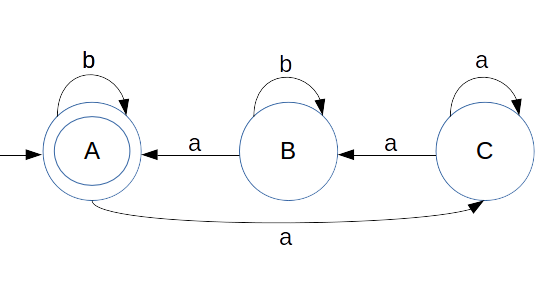## Conversion of Left Linear Grammar to Finite Automata

Converting left linear grammar to Finite Automata is simple.

1. Take reverse of CFG
2. Create Finite automata using privious example
3. Then again take reverse of the FA and that will be our final output
4. Start State: It will be the first production's state
5. Final State: Take those states which end up with input alphabets. eg. State A and C are below CFG

Here we are giving one right linear grammar
```			A -> Ba/Ab/b
B -> Ca/Bb
C -> Aa/Cb
```

#### We will follow the steps

1. Take the reverse of the CFG
2. ```			A -> aB/bA/b
B -> aC/bB
C -> aA/bC/a
```
3. Now create the FA
4.Note: Only state A is final state in this example
5. Now take reverse of the above FA (this is final output)
6.Note: Here state A was itself a final state that is why it again become start state as well as final state.